## Institute of Theoretical Computer Science

DMV - Fachgruppe Diskrete Mathematik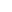Symposium Diskrete Mathematik 2004

Das Symposium der DMV Fachgruppe Diskrete Mathematik fand dieses Jahr am 7./8. Oktober 2004 an der ETH Zürich statt.

Während des Symposiums fand unter anderem die Bekanntgabe der Preisträger des Richard-Rado-Preis 2004, sowie die Preisübergabe statt.

Preisträger: Uli Wagner (links im Bild)
Ehrenvolle Anerkennung: Julian Pfeifle (rechts im Bild)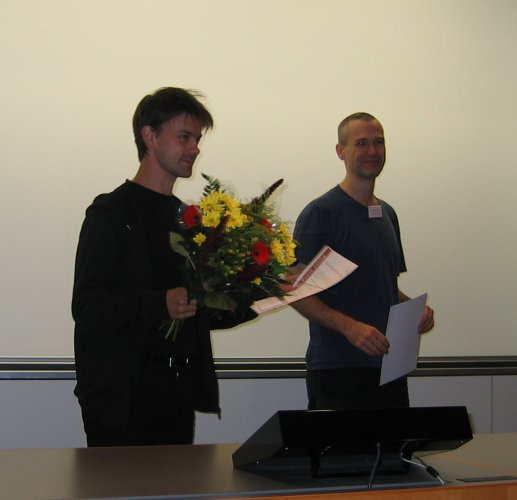Programm

Donnerstag, 7. Oktober 2004Noga Alon (Tel Aviv University) Probabilistic reasoning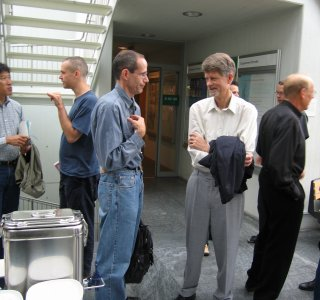KaffeepauseElmar Teufl (TU Graz) Oscillation and Iterative Functional EquationsMihyun Kang (HU Berlin) On evolution of random graph processes with degree restrictions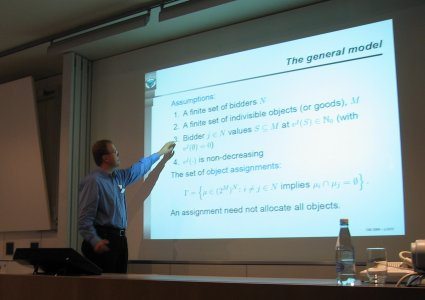Sven de Vries (TU München) Combinatorial Auctions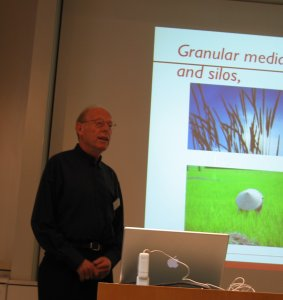Thomas Liebling (ETH Lausanne) "Are granular media a fourth state of matter? Distinct element simulation may provide some insight"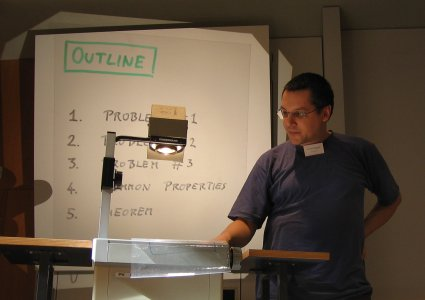Gerhard Woeginger (TU Eindhoven) Three problems and one theoremAbendessen im Dozentenfoyer der ETH

Freitag, 8. Oktober 2004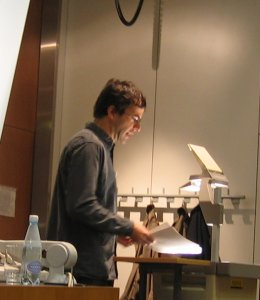Gabor Tardos (Hungarian Academy of Sciences, Budapest) Excluded 0-1 matricesKaffeepause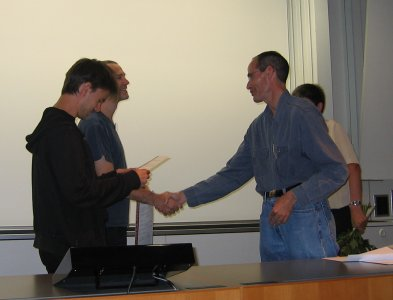Verleihung des Richard-Rado-PreisesUli Wagner (Hebrew University of Jerusalem) k-Sets, Crossing Numbers of Complete Graphs, and the Upper Bound Theorem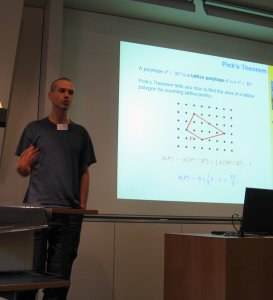Julian Pfeifle (IMUB, Barcelona) Coefficients and Roots of Ehrhart PolynomialsRobert Elsässer (Universität Paderborn) On Randomized Broadcasting in Large Networks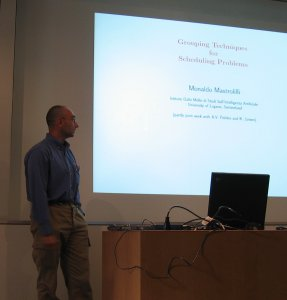Monaldo Mastrolilli (IDSIA, Manno-Lugano) Grouping Techniques for Scheduling ProblemsCarsten Thomassen (Technical University of Denmark, Kopenhagen) List colorings and the chromatic polynomial of a graph

Abstracts der Vorträge

 Speaker: Noga Alon (Tel Aviv University) Title: Probabilistic Reasoning

Abstract:

The discovery that deterministic statements can be proved by probabilistic reasoning, led already more than fifty years ago to several striking results in various mathematical disciplines. It soon became clear that the method, which is now called the probabilistic method, is a very powerful tool for proving results in Discrete Mathematics.

After a brief light example that illustrates the fact that probabilistic arguments may be counter-intuitive, I will describe some recent applications of probabilistic ideas in the design of efficient algorithms and in the proofs of combinatorial statements.

The main theme is that a probabilistic point of view may be very helpful even when we are interested only in purely deterministic algorithms, or in purely deterministic mathematical statements.

 Speaker: Elmar Teufl (TU Graz) Title: Oscillation and Iterative Functional Equations

Abstract:

In this talk we will present some asymptotic problems from combinatorics and probability theory which involves logarithmic oscillations. These problems originate from 2-3 trees, Galton-Watson processes and random walk on fractal-like graphs. For the presented results this specific type of asymptotic behaviour can be deduced from a careful analysis of the corresponding generating function. In fact it turns out that the generating function satisfies an iterative functional equation. This enables us to use ideas from complex dynamics and the method of singularity analysis.

 Speaker: Mihyun Kang (HU Berlin) Title: On evolution of random graph processes with degree restrictions

Abstract:

A random graph process is a Markov chain whose stages are graphs on n vertices. It starts with an empty graph on n vertices, and in each step a new graph is obtained from a current graph by adding a new edge according to a prescribed rule. A seminal example is the standard random graph process introduced by Erdös and Renyi.

Recently random graph processes with degree restrictions received much attention. We discuss how the connectivity of a graph changes and how the structure of components evolves as the number of edges grows.

 Speaker: Sven de Vries (TU München) Title: Combinatorial Auctions

Abstract:

Many auctions involve the sale of a variety of distinct assets. Examples are procurement of raw materials, spectrum slots, delivery routes and reinsurance contracts. Because of complementarities or substitution effects between the different assets, bidders have preferences (unobservable to the auctioneer) not just for particular items but for sets of items. The problem of finding the best allocation reduces to an optimization problem with incomplete information. We want to survey applications and approaches of combinatorial auctions.

 Speaker: Thomas Liebling (ETH Lausanne) Title: "Are granular media a fourth state of matter? Distinct element simulation may provide some insight"

Abstract:

Granular media are ubiquitous in nature and very important in technology. While they flow forming vortices at times like liquids do. Unlike these they form dunes. They can behave like gases or solids; they can segregate, homogenize and crystallize. While traditionally only physical experimentation was available for their study, today numerical simulation is becoming a powerful supplementary tool to describe and understand granular flows. We will survey work of our group at EPFL leading to the development of effective codes able to treat large populations of non-spherical grains in three dimensions. The main ingredients for distinct element simulation are appropriate choice of particle interaction models and contact detection procedures. The latter use tools from discrete, computational geometry, in particular generalized Delaunay triangulations.

 Speaker: Gerhard J Woeginger (TU Eindhoven) Title: Three problems and one theorem

Abstract:

The talk discusses three algorithmic problems (a weight balancing problem; a data management problem; a sequencing problem vaguely related to dartboards), some of their combinatorial properties, and one unifying theorem.

 Speaker: Gabor Tardos (Hungarian Academy of Sciences, Budapest) Title: Excluded 0-1 matrices

Abstract:

We say that a 0-1 matrix A contains another 0-1 matrix P if P is a submatrix of A or it can be obtained from a submatrix of A by switching some 1 entries to 0. How many 1 entries can an n by n 0-1 matrix have if it avoids one or more given patterns P?

This extremal problem can be regarded as a generalization of Turan-type extremal graph theory to graphs with an ordered vertex set. It also generalizes certain problems in the Davenport-Schinzel theory.

Besides reviewing recent results on the above problem, I mention a few (hopefully tractable) open problems.

 Speaker: Uli Wagner (Hebrew University of Jerusalem) Title: k-Sets, Crossing Numbers of Complete Graphs, and the Upper Bound Theorem

Abstract:

A k-set of a finite set of points in Rd is a subset of k points that can be separated from the remaining points by a hyperplane. The crossing number of a graph G is the smallest number of crossings between edges in any drawing of G in the plane.

We discuss connections between these two notions, and how they both relate to McMullen's famous theorem on the maximum number of faces of a d-dimensional convex polytope with a given number of vertices.

 Speaker: Julian Pfeifle (IMUB, Barcelona) Title: Coefficients and Roots of Ehrhart Polynomials

Abstract:

The Ehrhart polynomial of a convex lattice polytope P counts the number of integer points in integral dilates of P. We will discuss what information about P can be obtained by expanding the Ehrhart polynomial in different bases, and present new linear inequalities satisfied by the coefficients of Ehrhart polynomials. We then talk about the possible location of the roots of the Ehrhart polynomial in the complex plane, give new bounds on the location of the real zeros, and close with some conjectures and open problems.

 Speaker: Robert Elsässer (Universität Paderborn) Title: On Randomized Broadcasting in Large Networks

Abstract:

Broadcasting algorithms have a various range of applications in several fields of computer science. In this paper we study randomized broadcasting algorithms in different graph classes, which are often used to model large networks. First, we consider a modified version of the random phone call model of Karp et al. to show that the communication overhead can be reduced to O(n \ln \ln n) in almost all connected random graphs and this result also holds if the nodes do not know anything about the size of the network. Then, we consider an agent based broadcasting model and prove that, by eploiting the advantages of the distribution of the agents among the nodes of the graph, any information can almost always be completely distributed in a network obeying the scale-free power low degree distribution in O(D) steps, where D represents the diameter of the corresponding graph.

 Speaker: Monaldo Mastrolilli (IDSIA, Manno-Lugano) Title: Grouping Techniques for Scheduling Problems

Abstract:

We show that faster and simpler polynomial time approximation schemes for several scheduling problems can be obtained by reducing the number of jobs to a constant (that depends on the desired approximation error), and applying enumeration or dynamic programming afterwards. The reduced set of jobs is computed by first structuring the input and then grouping jobs that have the same structure. The idea appears attractive since it is easy to implement, fast and can be used as a preprocessing step in other algorithms (like branch and bound or cutting plane algorithms). We conclude the presentation with an empirical study.

 Speaker: Carsten Thomassen (Technical University of Denmark) Title: List colorings and the chromatic polynomial of a graph

Abstract:

The chromatic polynomial P(G,k) of a graph G is the number of vertex-colorings of G with k available colors. The chromatic polynomial was introduced by Birkhoff in 1912 in order to study the 4-Color Problem. Although the chromatic polynomial has not been very successful for coloring problems, it has been studied for several other reasons, primarily by mathematicians but also by physicists. In this talk, basic properties of the chromatic polynomial will be discussed, including a recent connection to the Hamiltonian cycle (travelling salesmans) problem.

List coloring is a more recent subject. Again, we want to color the vertices of the graph. But now every vertex v must receive a color from a prescribed list L(v) of available colors. We review some list color results and point out how list colorings can contribute to the chromatic polynomial.